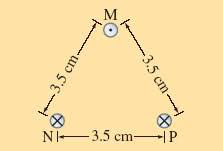# Magnetic Force of a copper wire

#### Kandycat

1. The problem statement, all variables and given/known data

The top wire is 1.00 mm diameter copper wire and is suspended in air due to the two magnetic forces from the bottom two wires. The current flow through the two bottom wires is 95 A in each. Calculate the required current flow in the suspended wire.2. Relevant equations
Force between two parallel wires is
F2= u0I1I2l2/(2*pi*d)

3. The attempt at a solution
Fm = Fnp
Fm = u0*95 A * 95 A * l2/(2*pi* .038 m)

But they do give me length...

#### LowlyPion

Homework Helper
1. The problem statement, all variables and given/known data

The top wire is 1.00 mm diameter copper wire and is suspended in air due to the two magnetic forces from the bottom two wires. The current flow through the two bottom wires is 95 A in each. Calculate the required current flow in the suspended wire.2. Relevant equations
Force between two parallel wires is
F2= u0I1I2l2/(2*pi*d)

3. The attempt at a solution
Fm = Fnp
Fm = u0*95 A * 95 A * l2/(2*pi* .038 m)

But they do give me length...
They do or do not give you length? (You don't need it.)

But you do need to develop the weight per unit length of the top wire from the volume of the copper and its density. m*g = ρ*v*g per unit length.

The force of the B field however is a vector that you need to resolve into the distances and angles from the 2 wires below that are supporting it.

Figure the angle to the vertical is 30° for an equilateral triangle in this configuration, so vertically you will have 2*F*cos30° as your upward force from the magnetic field where F is determined by the Force relationship for 2 || wires.

#### Kandycat

So are you saying that 2*F*cos30 = ρ*v*g?

#### LowlyPion

Homework Helper
So are you saying that 2*F*cos30 = ρ*v*g?
After a fashion I suppose, taking care to get the unit length accounted for.

You should draw out the vectors and satisfy yourself that the components add in this way.

### The Physics Forums Way

We Value Quality
• Topics based on mainstream science
• Proper English grammar and spelling
We Value Civility
• Positive and compassionate attitudes
• Patience while debating
We Value Productivity
• Disciplined to remain on-topic
• Recognition of own weaknesses
• Solo and co-op problem solving